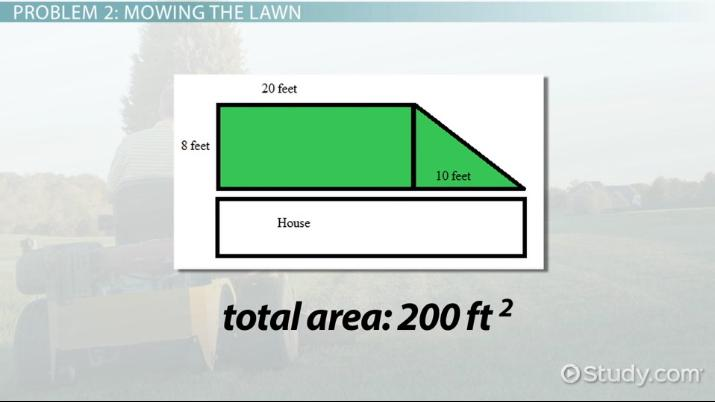Area: Real-World Geometry Problems

Lesson Transcript
Instructor: Elizabeth Foster

Elizabeth has been involved with tutoring since high school and has a B.A. in Classics.

Understanding how to calculate area is valuable to real-world situations like decorating a room or plotting a garden. Learn how geometry and area are applied in the real world, and see example problems using carpet and trampoline installation and mowing the lawn. Updated: 11/18/2021

Area Review

In this lesson, we're going to go over some real-world applications of area. But first, we'll get a quick refresher on what area is and how to calculate it.

Area is the amount of space covered by a two-dimensional shape.

To find the area of a square or rectangle, multiply the length times the width. To find the area of a circle, multiply pi times the radius squared. To find the area of a triangle, multiply one-half the base times the height. If you want to find the area of a more complicated shape, start by breaking it up into smaller parts.

Now, let's look at some real-world problems involving area.An error occurred trying to load this video.

Try refreshing the page, or contact customer support.

Coming up next: Perimeter: Real-World Geometry Problems

You're on a roll. Keep up the good work!

Replay
Your next lesson will play in 10 seconds
• 0:00 Area Review
• 0:42 Problem 1: Sue's Carpet
• 1:28 Problem 2: Mowing the Lawn
• 2:39 Problem 3: Trampolines
• 4:00 Lesson Summary
Save Save

Want to watch this again later?

Timeline
Autoplay
Autoplay
Speed Speed

Problem 1: Sue's Carpet

Sue is getting new carpet for her house. She can choose between two carpet installers. One will install any amount of carpet for a flat fee of $100. The other charges$0.85 per square foot. Sue's living room is 10 feet long and 14 feet wide. Which carpet installer should she choose?

Well, first we'll have to figure out how many square feet of carpet she needs, so we can tell what Installer B charges. Sue's living room is 10 feet long and 14 feet wide, so the area is

10 * 14 = 140 square feet

At $0.85 per square foot, that's$119 to install. So, Sue would be better-off going with the flat fee of $100, which would save her$19.

Problem 2: Mowing the Lawn

Saving $19 might not be huge money, but it would probably be appreciated by the hard-working protagonist of our next problem. Here it is: Mark's mother pays him to mow the lawn. Their lawn looks like this (see video). If Mark makes$0.05 per square foot of lawn, how many times will he have to mow the lawn to pay the $56 he needs to fill up his gas tank? You can see here that the lawn is basically a rectangle with a triangle stuck onto it at the end. The length of the rectangle is 20 feet, and the width is 8 feet. So, the area of just the rectangle part is 20 * 8 = 160 square feet The width of the rectangle is also the base of the triangle. The height of the triangle is 10 feet. So, the area of the triangular part is 1/2 * 8 * 10 = 40 feet 40 + 160 is 200, so the entire area of the lawn is 200 feet. At$0.05 per square foot, that's \$10 for mowing the lawn. To fill his tank, Mark would have to mow the lawn 6 times. At least it's a pretty small lawn!

Problem 3: Trampolines

A small lawn might be easy for Mark to mow, but it's not so wonderful for the next problem, which is all about how much stuff you can cram into the backyard.

To unlock this lesson you must be a Study.com Member.

Register to view this lesson

Are you a student or a teacher?